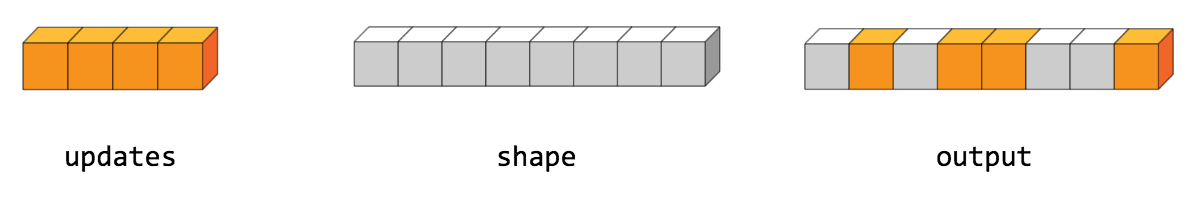# TensorScatterUpdate

public final class TensorScatterUpdate

Scatter `updates` into an existing tensor according to `indices`.

This operation creates a new tensor by applying sparse `updates` to the passed in `tensor`. This operation is very similar to `tf.scatter_nd`, except that the updates are scattered onto an existing tensor (as opposed to a zero-tensor). If the memory for the existing tensor cannot be re-used, a copy is made and updated.

If `indices` contains duplicates, then their updates are accumulated (summed).

WARNING: The order in which updates are applied is nondeterministic, so the output will be nondeterministic if `indices` contains duplicates -- because of some numerical approximation issues, numbers summed in different order may yield different results.

`indices` is an integer tensor containing indices into a new tensor of shape `shape`. The last dimension of `indices` can be at most the rank of `shape`:

indices.shape[-1] <= shape.rank

The last dimension of `indices` corresponds to indices into elements (if `indices.shape[-1] = shape.rank`) or slices (if `indices.shape[-1] < shape.rank`) along dimension `indices.shape[-1]` of `shape`. `updates` is a tensor with shape

indices.shape[:-1] + shape[indices.shape[-1]:]

The simplest form of scatter is to insert individual elements in a tensor by index. For example, say we want to insert 4 scattered elements in a rank-1 tensor with 8 elements.In Python, this scatter operation would look like this:

>>> indices = tf.constant([, , , ]) >>> updates = tf.constant([9, 10, 11, 12]) >>> tensor = tf.ones(, dtype=tf.int32) >>> print(tf.tensor_scatter_nd_update(tensor, indices, updates)) tf.Tensor([ 1 11 1 10 9 1 1 12], shape=(8,), dtype=int32)

We can also, insert entire slices of a higher rank tensor all at once. For example, if we wanted to insert two slices in the first dimension of a rank-3 tensor with two matrices of new values.

In Python, this scatter operation would look like this:

>>> indices = tf.constant([, ]) >>> updates = tf.constant([[[5, 5, 5, 5], [6, 6, 6, 6], ... [7, 7, 7, 7], [8, 8, 8, 8]], ... [[5, 5, 5, 5], [6, 6, 6, 6], ... [7, 7, 7, 7], [8, 8, 8, 8]]]) >>> tensor = tf.ones([4, 4, 4], dtype=tf.int32) >>> print(tf.tensor_scatter_nd_update(tensor, indices, updates).numpy()) [[[5 5 5 5] [6 6 6 6] [7 7 7 7] [8 8 8 8]] [[1 1 1 1] [1 1 1 1] [1 1 1 1] [1 1 1 1]] [[5 5 5 5] [6 6 6 6] [7 7 7 7] [8 8 8 8]] [[1 1 1 1] [1 1 1 1] [1 1 1 1] [1 1 1 1]]]

Note that on CPU, if an out of bound index is found, an error is returned. On GPU, if an out of bound index is found, the index is ignored.

### Public Methods

 Output asOutput() Returns the symbolic handle of a tensor. static TensorScatterUpdate create(Scope scope, Operand tensor, Operand indices, Operand updates) Factory method to create a class wrapping a new TensorScatterUpdate operation. Output output() A new tensor with the given shape and updates applied according to the indices.

## Public Methods

#### public Output<T> asOutput()

Returns the symbolic handle of a tensor.

Inputs to TensorFlow operations are outputs of another TensorFlow operation. This method is used to obtain a symbolic handle that represents the computation of the input.

#### public static TensorScatterUpdate<T> create(Scope scope, Operand<T> tensor, Operand<U> indices, Operand<T> updates)

Factory method to create a class wrapping a new TensorScatterUpdate operation.

##### Parameters
scope current scope Tensor to copy/update. Index tensor. Updates to scatter into output.
##### Returns
• a new instance of TensorScatterUpdate

#### public Output<T> output()

A new tensor with the given shape and updates applied according to the indices.

[{ "type": "thumb-down", "id": "missingTheInformationINeed", "label":"Missing the information I need" },{ "type": "thumb-down", "id": "tooComplicatedTooManySteps", "label":"Too complicated / too many steps" },{ "type": "thumb-down", "id": "outOfDate", "label":"Out of date" },{ "type": "thumb-down", "id": "samplesCodeIssue", "label":"Samples / code issue" },{ "type": "thumb-down", "id": "otherDown", "label":"Other" }]
[{ "type": "thumb-up", "id": "easyToUnderstand", "label":"Easy to understand" },{ "type": "thumb-up", "id": "solvedMyProblem", "label":"Solved my problem" },{ "type": "thumb-up", "id": "otherUp", "label":"Other" }]